Chapter 5 - DerivativesCustom SearchCHAPTER 5 DERIVATIVES LEARNING OBJECTIVES Upon completion of this chapter, you should be able to do the following: 1. Compute the derivative of a constant. 2. Compute the derivative of a variable raised to a power. 3. Compute the derivative of the sum and product of two or more functions and the quotient of two functions. 4. Compute the derivative of a function raised to a power, in radical form, and by using the chain rule. 5. Compute the derivative of an inverse function, an implicit funcŁtion, a trigonometric function, and a natural logarithmic function. 6. Compute the derivative of a constant raised to a variable power. INTRODUCTION In the previous chapter on limits, we used the delta process to find the limit of a function as Ax approached zero. We called the result of this tedious and, in some cases, lengthy process the derivative. In this chapter we will examine some rules used to find the derivative of a function without using the delta process. To find how y changes as x changes, we take the limit of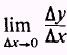which is called the derivative of y with respect to x; we use the symbol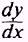to indicate the derivative and write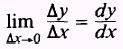In this section we will learn a number of rules that will enable us to easily obtain the derivative of many algebraic functions. In the derivation of these rules, which will be called theorems, we will assume thator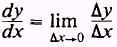exists and is finite. DERIVATIVE OF A CONSTANT The method we will use to find the derivative of a constant is similar to the delta process used in the previous chapter but inŁcludes an analytical proof. A diagram is used to give a geometrical meaning of the function. Theorem 1. The derivative of a constant is zero. Expressed as a formula, this may be written as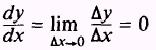where y = c. PROOF: In figure 5-1, the graph of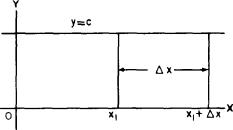Figure 5-1.-Graph of y = c, where c is a constant. y=c where c is a constant, the value of y is the same for all values of x, and any change in x (that is,x) does not affect y; then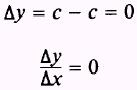and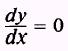Another way of stating this is that when x is equal to x1 and when x is equal to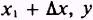has the same value. Therefore, y=c and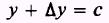so thatand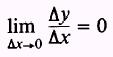ThenThe equation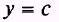represents a straight line parallel to the X axis. The slope of this line will be zero for all values of x. Therefore, the derivative is zero for all values of x. EXAMPLE. Find the derivative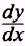of the function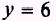SOLUTION: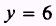and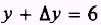Therefore,Integrated Publishing, Inc. - A (SDVOSB) Service Disabled Veteran Owned Small Business